SELF 44 S.B. Karavashkin and O.N. KaravashkinaTrue, the experiments ‘without crystals’ have been conducted with electrons also. “The first experiments corroborating the de Broglie hypothesis have been carried out on crystals that are most convenient diffraction lattice created by nature to observe the diffraction of electron waves. Further, a number of diffraction experiments similar to the classical experiments that are well known in optics has been done with electrons. Diffraction of electrons has been observed at the edge of semi-finite plane, on two splits and so on. The experiments have been done on the diffraction of electrons in a heterogeneous electric field that took part of an analogue of Fresnel’s bi-prism. All these experiments corroborated the presence of wave properties in electrons” . In particular, “to reveal, whether the individual particle has wave properties, the team of our physicists led by V.A. Fabricant did in 1949 the diffraction study with a very weak beam of electrons. In these experiments, the time interval between two sequential passes of electrons through the crystal was 30 000 times larger than the time during which one electron passed the whole instrument. Thus, electrons diffracted in the crystal one by one; their interaction with each other as the cause producing the diffraction pattern was fully excluded. The qualitative appearance of distribution of the diffracted electrons in the photographic plate is shown in Fig. 3. When short duration of the experiment, the points on the plate related to the hits of electrons are distributed occasionally (Fig. 3a). But when quite long duration of the experiment, the distribution of points gains the shape of concentric rings (Fig. 3b) typical for diffraction on a poly-crystal. Thus, it was proven that the wave properties are inherent in a single electron” .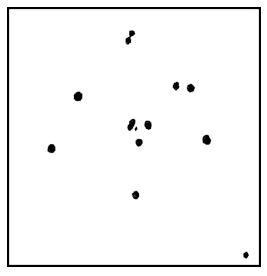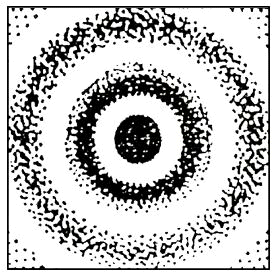a                                                                                     b
 Fig. 3. Distribution of diffracted electrons in the photographic plate: a – when short-duration experiment, b – in case of long-duration experiment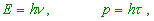(1)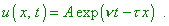(2)
 This wave propagates along the axis x with a definite speed – the phase speed u” [2, p. 109]. Thus, according to de Broglie, a particle has to gain the properties of monochromatic coherent wave and to lose its finite geometric size, and not only at the moment when it interacts with the substance but generally. When the electron flies to the target, it can ‘feel’ this target, only having approached it immediately; it would be illogic, this electron to have finite size before interaction with the target, at the moment of interaction to turn into an infinite wave and after the interaction to become again a particle of quantum size. But if the electron itself is an infinite wave (or the probability to reveal it at some or other point of space after Born [2, p. 115] obeys the regularity (2), which is similar), such widespread measurements as the dilation time of turning on the current, the flight time of electron in vacuum tubes etc. would lose their logic. The probability to find an electron along whole measured interval would relate not to the period comparable with the frequency of X-rays along the whole infinite interval, anyway in limits much larger than the size of lab instruments, nothing to say of dilation time to pass a limited interval. Just so from the very beginning of quantum mechanic formation the de Broglie wave was understood as a chain of waves. In particular, Fermi, when solved the Schroedinger equation for a point on an infinite line (the model of free electron) and encountered the disappearance of wave function discreteness, expressed, after other supporters of photon theory, the following premise: “Let there are no abrupt discrete energetic levels but instead them are ‘blurred’ levels corresponding to ‘blurring’ the wave function u (x) across the interval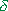k around the point k = k0 , i.e. the function u is represented as a ‘wave packet’ (see Fig. 4):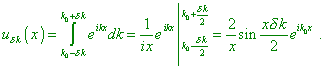(3)
 Such solution already can be normalised with very smallk . Then it corresponds to almost definite values of energy” [6, p. 31].Fig. 4. The ‘wave packet’ for the function   uk  [6, p. 31]
 But this is not a real solution of the problem. First, the function (3) and the de Broglie function (2) are basically different. Second, the wave chain in (3) has a spectrum, and noting the small size of electron, the spectrum will be vast and it cannot be limited by a small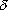k , as Fermi supposed. Not in vain in the footnote to this Fermi’s derivation, where he referred that in the further lectures he will discuss the problems of energy of such packet, the publishers marked: “In the synopsis of related (13th) lecture the direct discussion of the issue of energy of such packet is absent. – Publishers” [6, p. 31]. The matter is that, third, “In the beginning, Schroedinger has undertook an attempt to interpret the corpuscles, in particular electrons, as wave packets. Although the Schroedinger formulas are absolutely correct (? – Authors), none the less, his interpretation could not stand. Actually, on one hand, … , wave packets blur in time. On the other, describing the interaction of two electrons as an interaction of two wave packets in a trivial 3-D space encounters insurmountable difficulties. It was Born who suggested the today conventional interpretation. From the point of this interpretation, the whole course of events in the physical system is determined by the laws of probability. Then to such or other position of a particle in space there will correspond some probability determined by the de Broglie wave associated with the state of particle. Thus, the mechanical process is entailed with the wave process – the process of propagation of probability wave. This last obeys the Schroedinger equation whose importance is to determine the probability of any variant of the course of events in the mechanical process. If, for example, at some point of space the probability wave has zero amplitude, this means that the probability to reveal an electron at this point is infinitesimal” [2, p. 114–115].